# Aquarium Calculator

Created by Hanna Pamuła, PhD candidate
Reviewed by Bogna Szyk
Last updated: Jul 30, 2022

Determining fish tank volume has never been easier - with this aquarium calculator you can do it in a blink of an eye. Find water volume 💧 which will fill up your container: choose between 7 typical aquarium shapes - from a simple box, through cylindrical variations, to bow front tanks. If you're wondering what're the formulas to calculate the aquarium size or you're not sure how to use this tool, just scroll down and you'll find the answer below.

If you're looking for the amount of water that goes into a swimming pool, check out our pool calculator. We also have a pond calculator if you're interested in backyard ponds.

## Aquarium shapes

In our aquarium calculator, we implemented seven popular fish tank shapes:

Name Aquarium shape

Rectangular prism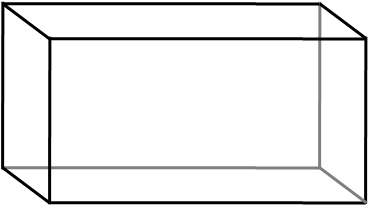Cube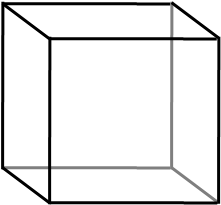Bow front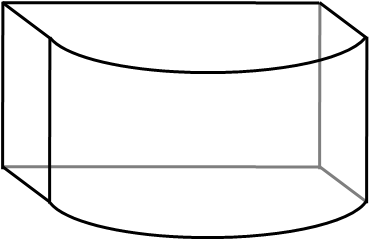Corner bow front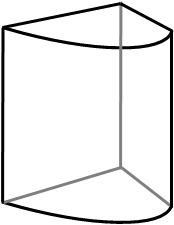Cylinder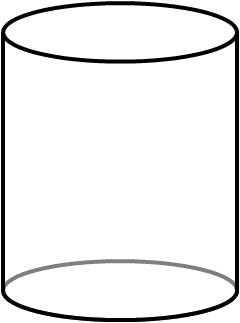Half cylinder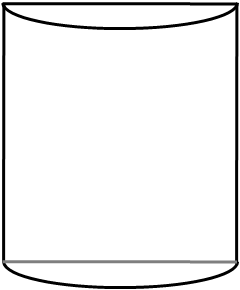Quarter cylinder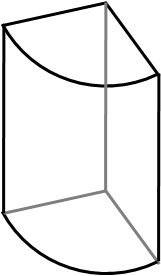## Formulas behind the fish tank calculator

If you want to calculate the aquarium size manually, you can use the formulas for volume depending on tank shape:

• Rectangular prism

To find the volume of a standard aquarium, calculate the volume of a rectangular prism (called also the volume of a box):

rectangular = height * width * length

• Cube

Cube-shaped aquarium has the easiest volume equation - simply raise the edge length to the third power:

cube = length³

• Cylinder and its fractions

If you want to calculate the water volume of a cylindrical aquarium, use the formula for cylinder volume:

cylinder = π * (diameter / 2)² * height

Your fish tank is a half-cylinder? No problem, just divide the formula above by 2:

half_cylinder = π * (diameter / 2)² * height / 2

To determine the volume of the quarter of the cylinder, take a fourth part of the full volume:

quarter_cylinder = π * radius² * height / 4

It's handier to use radius instead of diameter in this last case: diameter = 2 * radius.

• Bow front and bow front corner aquarium

Calculating the volume of the bowfront aquarium is a bit trickier. You need to have given four dimensions: height, width, length, and the full width of the aquarium, and then you can derive the final formula:

bowfront = height * width * length + 0.5 * r² * (α - sin(α)) * height

where α is a central angle of the circular segment, in radians and r is a radius of a circle.
α may be calculated as α = 2π - 4β, where β is an angle expressed as tan(β) = 0.5 * length / (full_width - width).

For the aquarium with bow front intended to stand in the corner, the formula is:

corner_bowfront = (0.5r² * (α - sin(α)) * height)/2

Aquarium in custom or bizarre shape? Check out our other tools such as triangular prism volume or spherical cap calculator which can find water volume for spherical aquariums, also called fish bowls. Just remember that such tanks are not appropriate for keeping fish and in some countries they're even forbidden! Spherical aquariums are usually small tanks, fish have to swim in circles, which is unnatural for them, and it's hard to put a filter into such a tank. Consider a different aquarium shape despite the fancy look of such a tank.

## How to use this tool as an aquarium gallon calculator

If you want to calculate how many gallons of water your tank contains, follow these simple steps:

1. Choose the shape of your aquarium. Assume that you have a bow front fish tank, so select this option from a drop-down list.

2. Enter the tank dimensions. In our case, we need to type four values. Let's say that the length is 35 in, full width - 18 in, width - 15 in, and height 20 in.

3. The aquarium calculator finds the volume of your tank. In our case, it's 51.55 gallons.

4. If you're wondering how much is that in liters, click on the unit name and select the one you're interested in - e.g., our aquarium volume in liters is 195.14.

Remember that those calculations may serve as estimations and the result obtained doesn't mean that precisely 51.55 gallons will fit into your tank. We don't take into account e.g. the thickness of the glass or the volume of other objects, such as sand, gravel, filter, plants and... fish. Also, you don't fill up your container completely, do you?

## Have a look at another example: how big is a 75-gallon aquarium?

You heard that your friend has just bought a 75-gallon aquarium. Well, it's hard to imagine, how big is that? Let's check it with this aquarium calculator!

1. Choose the fish tank shape. We know that our friend is rather a traditionalist, so his obvious choice is a standard rectangular prism tank.

2. Type the volume - in our case, put 75 gallons into the volume box.

As the given volume may be a result of an infinite combination of the rectangular prism dimensions, we need to put more information into:

3. Enter two tank dimensions. The usual height of the aquarium is something between 18-26 in, let's say it's 22 in. Additionally, assume the width is the same as the height.

4. We found out that this 75-gallon aquarium has ~35.8 in length, so the dimensions are 35.8 x 22 in x 22 in. Quite big, huh? Experiment with different dimensions and shapes to get a better understanding of the concept of volume.

## FAQ

### How do I calculate the volume of an aquarium?

The volume of an aquarium is the same as of any other object. You require the dimensions to estimate the volume.
The length, width, and height are the perimeters on which the volume of most shapes depends, except for cylindrical shapes. They require the diameter or radius to estimate the capacity of the object.

### Does shape of the aquarium impact its volume?

Yes, it does. Every shape has a different volume. And most of them have a different formula to calculate the volume.
For instance, the volume of a rectangular shape depends on the length, width, and height, or a cube needs only length, whereas cylindrical objects require diameter and length to estimate the volume.

### How many fish can I put in my 50 liter aquarium?

The number of fish you can put in 50l aquarium is 16 - 17. The general estimation is 1 liter of water for 1 centimeter of fish.

Suppose your fishes are 3 cm in size. Follow the steps to count the number of fish:

1. Note down the volume of your aquarium in liters;
2. Estimate the size of your fish in centimeters;
3. Divide the volume by the size; and
4. The result is the number of fish.

So, 50/3= 16.66, round it to 17 because you can't have half a fish.

### How many gallons of water can a 3m³ aquarium hold?

A 3m³ aquarium can hold 792.5 gallons of water, which is equal to 3000 liters. The m³, gallons, and liters are all units to measure the volume of a container.

1 m³ = 1000 liters

and

1 liter = 0.264172 US gallons

Hanna Pamuła, PhD candidate
Tank shape
rectangular prism
Dimensions
Length
in
Width
in
Height
in
Volume
Volume
US gal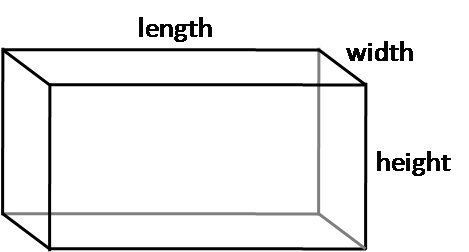People also viewed…

### Christmas tree

Welcome to the Christmas tree calculator, where you will find how to decorate your Christmas tree in the best way. Take a look at the perfect Christmas tree formula prepared by math professors and improved by physicists. Plan in advance how many lights and decorations you'll need!

### Crop factor

The crop factor calculator tells you the effective focal length and aperture f-stop of your camera in terms of standard 35mm lenses.

### Op-amp gain

Omni's op-amp gain calculator can help you to determine the voltage gain of an operational amplifier.

### Snowman

The perfect snowman calculator uses math & science rules to help you design the snowman of your dreams!